# JEE Main Mathematics Mock

## 25 Questions MCQ Test Mock Test Series for JEE Main & Advanced 2022 | JEE Main Mathematics Mock

Description
Attempt JEE Main Mathematics Mock | 25 questions in 60 minutes | Mock test for JEE preparation | Free important questions MCQ to study Mock Test Series for JEE Main & Advanced 2022 for JEE Exam | Download free PDF with solutions
QUESTION: 1

Solution:
QUESTION: 2

Solution:
QUESTION: 3

### The limiting point of the system of co-axial circles x2+y2-6x-6y+4=0, x2+y2-2x-4y+3=0 is

Solution:
QUESTION: 4

The are (in square units) of the region bounded by x2 = 8 y , x = 4 and x-axis is

Solution:
QUESTION: 5

The number of tangents drawn from the point (-1,2) to x2y2+2x-4y+4=0 is

Solution:

The correct option is Option D.

It is found that the centre of the circle is (-1,2)

Hence, no tangents would pass through it.

QUESTION: 6

The conjugate of complex number [(2+5i)/(4-3i)] is

Solution:
QUESTION: 7

[(1-i)/(1+i)] =

Solution:
QUESTION: 8

The solution of differential equation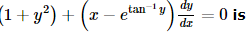Solution:
QUESTION: 9

The maximum value of the function f(x)=[(x)/(4+x+x2)] at the interval [-1,1] will be

Solution:
QUESTION: 10

sin⁻1 (cos(sin⁻1 x) + cos⁻1 (sin(cos⁻1 x) is equal to

Solution:
QUESTION: 11

In the following question, a Statement of Assertion (A) is given followed by a corresponding Reason (R) just below it. Read the Statements carefully and mark the correct answer-
Assertion(A): y = sin (ax + b) is a general solution of y" + a^2y = 0 .
Reason(R) : y = sin (ax + b)  is a trigonometric function.

Solution:
QUESTION: 12

The inverse of the converse of a conditional statement is the _______________.

Solution:

The inverse will insert NOT into the statement.
The converse will switch the IF and THEN.
The contrapositive does both.
The contrapositive is the inverse of the converse of the conditional statement.

QUESTION: 13

In the following question, a Statement of Assertion (A) is given followed by a corresponding Reason (R) just below it. Read the Statements carefully and mark the correct answer-
Assertion(A): The domain of the function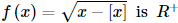Reason (R): The domain of the function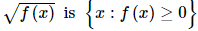Solution:
QUESTION: 14

If A is a square matrix such that A2 = I, then A⁻1 is equal to

Solution:
QUESTION: 15

Two finite sets have m and n elements, the total number of subsets of the first set is 56 more than the total number of subsets of the second. The value of m and n are respectively

Solution:

Let A denote the first set and B denote the second set
We have, n(A) = 2m and n(B) = 2n
As per the question, we have
n(A) = 56 + n(B)
⇒ n(A) - n(B) = 56
⇒ 2m - 2n = 56
⇒ 2n (2m - n - 1)
⇒ 2n (2m - n - 1) = 8 x 7
⇒ 2n = 8 = 23 or (2m - n - 1) = 7
⇒ n = 3 or 2m - n = 8 = 23 = 26 - 3
⇒ n = 3 or m - n = 3
⇒ n = 3 or m = 6
Hence, the required values of m and n are 6 and 3 respectively

QUESTION: 16

The equation of the directrix to the parabola y2 − 2x − 6y − 5 = 0 is

Solution:
QUESTION: 17

A die is thrown 100 times. If getting an odd number is considered a success, the variance of the number of successes is

Solution:
QUESTION: 18

If in a moderately asymmetrical distribution mode and mean of the data are 6λ and 9λ respectively, then median is

Solution:
QUESTION: 19

The sides of a parallelogram are lx + ny + n = 0, lx + my + n' = 0, mx + ly + n = 0, mx + ly + n' = 0, the angle between its diagonals is

Solution:
QUESTION: 20
Equation of normal to the curve y = x(2 - x) at the point (2, 0) is
Solution:
*Answer can only contain numeric values
QUESTION: 21

Let f : (–3, 3) → R be a differentiable function with f(0) = – 2 and f'(0) = – 1 and g(x) = (f(3f(x) + 6))then g'(0) is equal to.

Solution:
*Answer can only contain numeric values
QUESTION: 22

It is given that events A and B of an experiment are such that P(A / B) = 1 / 4 and P (B / A) = 1/12 and chance of occurence of an event "only A" is 1/12  (where P(x) denote the probability of occurence of event 'x'). Value of 22P(B) equals

Solution: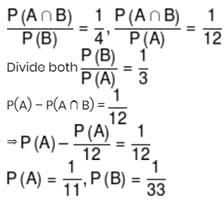*Answer can only contain numeric values
QUESTION: 23

At present a firm is manufacturing 2000 items. It is estimated that the rate of change of production P w.r.t. additional number of workers x is given by dP/dx = 100 - 12√x. If the firm employs 25 more workers, then the new level of production of items is:

Solution:
*Answer can only contain numeric values
QUESTION: 24

If the function ƒ(x) = 2 + x2 – e–x and g(x) = ƒ–1(x), then the value of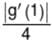equals

Solution:

g(ƒ(x)) = x ⇒ g'(ƒ(x)). ƒ'(x) = 1
ƒ(x) = 1 ⇒ x = 0
g'(1). ƒ'(0) = 1
ƒ'(x) = 2x + e–x
ƒ'(0) = 1
g'(1) = 1

*Answer can only contain numeric values
QUESTION: 25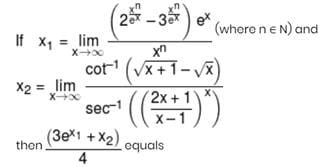Solution: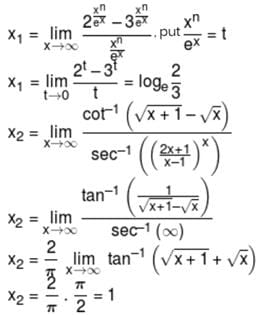Use Code STAYHOME200 and get INR 200 additional OFF Use Coupon Code